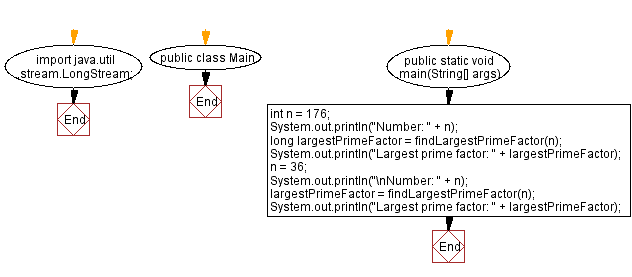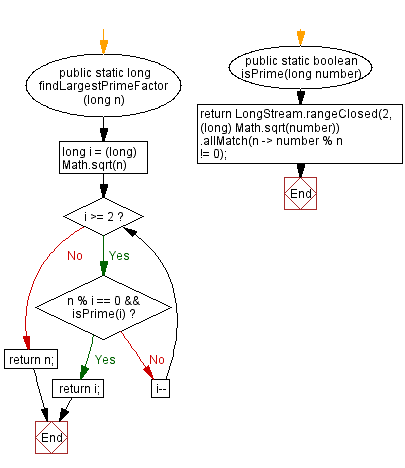# Java Program: Lambda expression to find largest prime factor

## Java Lambda Program: Exercise-24 with Solution

Write a Java program to implement a lambda expression to find the largest prime factor of a given number.

Sample Solution:

Java Code:

``````import java.util.stream.LongStream;

public class Main {
public static void main(String[] args) {
int n = 176;
System.out.println("Number: " + n);

long largestPrimeFactor = findLargestPrimeFactor(n);
System.out.println("Largest prime factor: " + largestPrimeFactor);
n = 36;
System.out.println("\nNumber: " + n);

largestPrimeFactor = findLargestPrimeFactor(n);
System.out.println("Largest prime factor: " + largestPrimeFactor);
}

public static long findLargestPrimeFactor(long n) {
for (long i = (long) Math.sqrt(n); i >= 2; i--) {
if (n % i == 0 && isPrime(i)) {
return i;
}
}
return n;
}

public static boolean isPrime(long number) {
return LongStream.rangeClosed(2, (long) Math.sqrt(number))
.allMatch(n -> number % n != 0);
}
}
``````

Sample Output:

```Number: 176
Largest prime factor: 11

Number: 36
Largest prime factor: 3
```

Explanation:

Main() method:

• Initializes a variable n with a value.
• Calls the findLargestPrimeFactor() method to calculate the largest prime factor of n.
• Prints the largest prime factor.

findLargestPrimeFactor(long n) method :

• It takes a long integer n as input.
• It iterates from the square root of n (converted to a long) downwards to 2.
• Inside the loop, it checks if n is divisible by the current value of i and if i is a prime number by calling the isPrime method.
• If both conditions are satisfied, it returns i, which is the largest prime factor.
• If no prime factors are found, it returns the original number n.

isPrime(long number):

• It takes a long integer number as input.
• It uses a LongStream to generate a range of numbers from 2 to the square root of number, inclusive.
• It checks if all numbers in the range satisfy the condition that number is not divisible evenly by any of them.
• If all numbers in the range satisfy the condition, it returns true, indicating that number is a prime number.
• If any number in the range divides number evenly, it returns false.

Flowchart:Live Demo:

Java Code Editor:

Improve this sample solution and post your code through Disqus

Java Lambda Exercises Previous: Lambda expression to find average string length.
Java Lambda Exercises Next: Lambda expression to convert integer to binary.

What is the difficulty level of this exercise?

Test your Programming skills with w3resource's quiz.

﻿

## Java: Tips of the Day

How do I remove repeated elements from ArrayList?

If you don't want duplicates in a Collection, you should consider why you're using a Collection that allows duplicates. The easiest way to remove repeated elements is to add the contents to a Set (which will not allow duplicates) and then add the Set back to the ArrayList:

```Set<String> set = new HashSet<>(yourList);
yourList.clear();